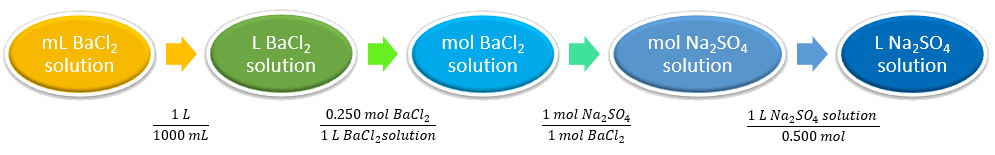# 10.3.3: Solution Stoichiometry

•• Anonymous
• LibreTexts
$$\newcommand{\vecs}{\overset { \rightharpoonup} {\mathbf{#1}} }$$ $$\newcommand{\vecd}{\overset{-\!-\!\rightharpoonup}{\vphantom{a}\smash {#1}}}$$$$\newcommand{\id}{\mathrm{id}}$$ $$\newcommand{\Span}{\mathrm{span}}$$ $$\newcommand{\kernel}{\mathrm{null}\,}$$ $$\newcommand{\range}{\mathrm{range}\,}$$ $$\newcommand{\RealPart}{\mathrm{Re}}$$ $$\newcommand{\ImaginaryPart}{\mathrm{Im}}$$ $$\newcommand{\Argument}{\mathrm{Arg}}$$ $$\newcommand{\norm}{\| #1 \|}$$ $$\newcommand{\inner}{\langle #1, #2 \rangle}$$ $$\newcommand{\Span}{\mathrm{span}}$$ $$\newcommand{\id}{\mathrm{id}}$$ $$\newcommand{\Span}{\mathrm{span}}$$ $$\newcommand{\kernel}{\mathrm{null}\,}$$ $$\newcommand{\range}{\mathrm{range}\,}$$ $$\newcommand{\RealPart}{\mathrm{Re}}$$ $$\newcommand{\ImaginaryPart}{\mathrm{Im}}$$ $$\newcommand{\Argument}{\mathrm{Arg}}$$ $$\newcommand{\norm}{\| #1 \|}$$ $$\newcommand{\inner}{\langle #1, #2 \rangle}$$ $$\newcommand{\Span}{\mathrm{span}}$$$$\newcommand{\AA}{\unicode[.8,0]{x212B}}$$

##### Learning Objectives
• Determine amounts of reactants or products in aqueous solutions.

As we learned previously, double replacement reactions involve the reaction between ionic compounds in solution and, in the course of the reaction, the ions in the two reacting compounds are “switched” (they replace each other). Because these reactions occur in aqueous solution, we can use the concept of molarity to directly calculate the number of moles of reactants or products that will be formed, and therefore their amounts (i.e. volume of solutions or mass of precipitates).As an example, lead (II) nitrate and sodium chloride react to form sodium nitrate and the insoluble compound, lead (II) chloride.

$\ce{Pb(NO_3)_2 (aq) + 2 NaCl (aq) → PbCl_2 (s) + 2 NaNO3 (aq)} \label{EQ1}$

In the reaction shown above, if we mixed 0.123 L of a 1.00 M solution of $$\ce{NaCl}$$ with 1.50 M solution of $$\ce{Pb(NO3)2}$$, we could calculate the volume of $$\ce{Pb(NO3)2}$$ solution needed to completely precipitate the $$\ce{Pb^{2+}}$$ ions.

The molar concentration can also be expressed as the following:

$1.00\,M \,\ce{NaCl} = \dfrac{1.00 \; mol \; \ce{NaCl}}{1 \; L \; \ce{NaCl} \; \text{solution}} \nonumber$

and

$1.50\,M\, \ce{Pb(NO3)2} = \dfrac{1.50 \; mol \; \ce{Pb(NO_3)_2}}{1 \; L \; \ce{Pb(NO_3)_2} \text{solution}} \nonumber$

First, we must examine the reaction stoichiometry in the balanced reaction (Equation \ref{EQ1}). In this reaction, one mole of $$\ce{Pb(NO3)2}$$ reacts with two moles of $$\ce{NaCl}$$ to give one mole of $$\ce{PbCl2}$$ precipitate. Thus, the concept map utilizing the stoichiometric ratios is:so the volume of lead (II) nitrate that reacted is calculated as:

$0.123 \; L \; \ce{NaCl} \, \text{solution} \times \dfrac{1.00 \; mol \; \ce{NaCl}}{1 \; L \; \ce{NaCl} \; \text{solution}} \times \dfrac{1 \; mol \; \ce{Pb(NO_3)_2}}{2 \; mol \; \ce{NaCl}} \times \dfrac{1 \; L \; \ce{Pb(NO_3)_2} \; \text{solution}}{1.5 \; mol \; \ce{Pb(NO_3)_2}} = 0.041 \; \ce{Pb(NO_3)_2}\,L \; \text{solution} \nonumber$

This volume makes intuitive sense for two reasons: (1) the number of moles of $$\ce{Pb(NO3)2}$$ required is half of the number of moles of $$\ce{NaCl}$$, based off of the stoichiometry in the balanced reaction (Equation \ref{EQ1}); (2) the concentration of $$\ce{Pb(NO3)2}$$ solution is 50% greater than the $$\ce{NaCl}$$ solution, so less volume is needed.

##### Example $$\PageIndex{1}$$

What volume (in L) of 0.500 M sodium sulfate will react with 275 mL of 0.250 M barium chloride to completely precipitate all $$\ce{Ba^{2+}}$$ in the solution?

###### Solution
Solutions to Example 13.8.1
Steps for Problem Solving Example $$\PageIndex{1}$$
Identify the "given" information and what the problem is asking you to "find."

Given: 275 mL BaCl2

0.250 M $$\ce{BaCl2}$$ or $$\displaystyle \dfrac{0.250\; mol BaCl_2}{1\; L\; BaCl_2\; solution}$$

0.500 M $$\ce{Na2SO4}$$ or $$\displaystyle \dfrac{0.500\; mol Na_2SO_4}{1\; L\; Na_2SO_4\; solution}$$

Find: Volume $$\ce{Na2SO4}$$ solution.

Set up and balance the chemical equation.

$$\ce{Na2SO4(aq) + BaCl2(aq) -> BaSO4(s)} + \underline{2} \ce{NaCl (aq)}$$

An insoluble product is formed after the reaction.

List other known quantities.

1 mol of Na2SO4 to 1 mol BaCl2

1000 mL = 1 L

Prepare a concept map and use the proper conversion factor.Cancel units and calculate. $$\displaystyle 275\cancel{mL \; BaCl_2 \; solution}\times \dfrac{1\cancel{L}}{1000\cancel{mL}}\times \dfrac{0.250 \cancel{mol \; BaCl_2}}{1 \cancel{L\;BaCl_2 \; solution}}\times \dfrac{1 \cancel{mol \; Na_2SO_4}}{1 \cancel{mol \; BaCl_2}}\times \dfrac{1\; L \; Na_2SO_4 \; solution}{0.500 \cancel{mol Na_2SO_4}}$$ = 0.1375 L sodium sulfate
Think about your result. The lesser amount (almost half) of sodium sulfate is to be expected as it is more concentrated than barium chloride. Also, the units are correct.
##### Exercise $$\PageIndex{1}$$

What volume of 0.250 M lithium hydroxide will completely react with 0.500 L of 0.250 M of sulfuric acid solution?

0.250 L $$\ce{LiOH}$$ solution